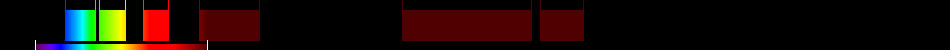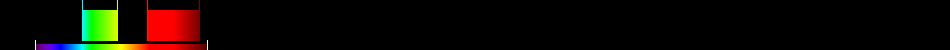# Information for Sensor and Index

## Formula

General Formula $\frac{{\mathrm{NDVI}}+0,5}{|{\mathrm{NDVI}}+0,5|}·\sqrt{|\left({\mathrm{NDVI}}\right)+0,5|}$ $\frac{\left(\frac{{3}-{2}}{{3}+{2}}\right)+0,5}{|\left(\frac{{3}-{2}}{{3}+{2}}\right)+0,5|}·\sqrt{|\left(\left(\frac{{3}-{2}}{{3}+{2}}\right)\right)+0,5|}$ Automatic

## Sensor

Name Resource21 Resource21 6 450-1650 10-100 98 Resource21 1999-00-00 Off-Nadir Repeat Cycle: Twice in 25 min (with 4 satellites) yes

## Index

Name Corrected Transformed Vegetation Index CTVI $\frac{{\mathrm{NDVI}}+0,5}{|{\mathrm{NDVI}}+0,5|}·\sqrt{|\left({\mathrm{NDVI}}\right)+0,5|}$ 490:570,640:760 Derived from specific sensor formula

## References